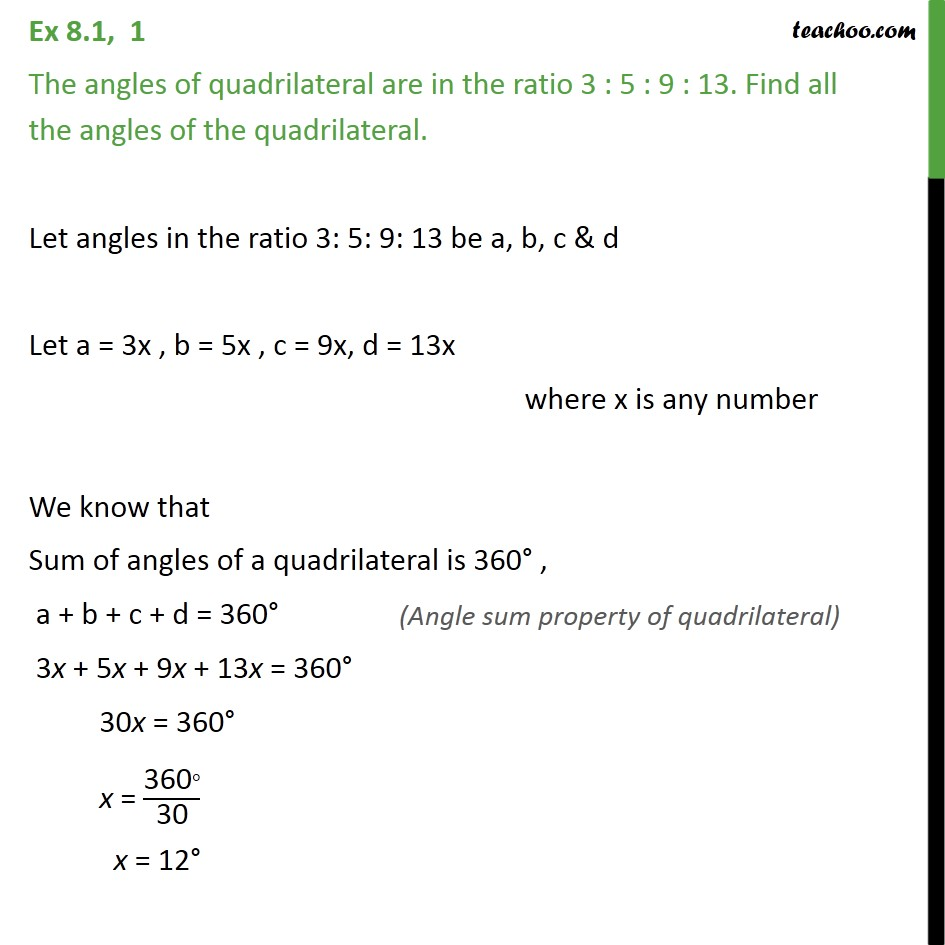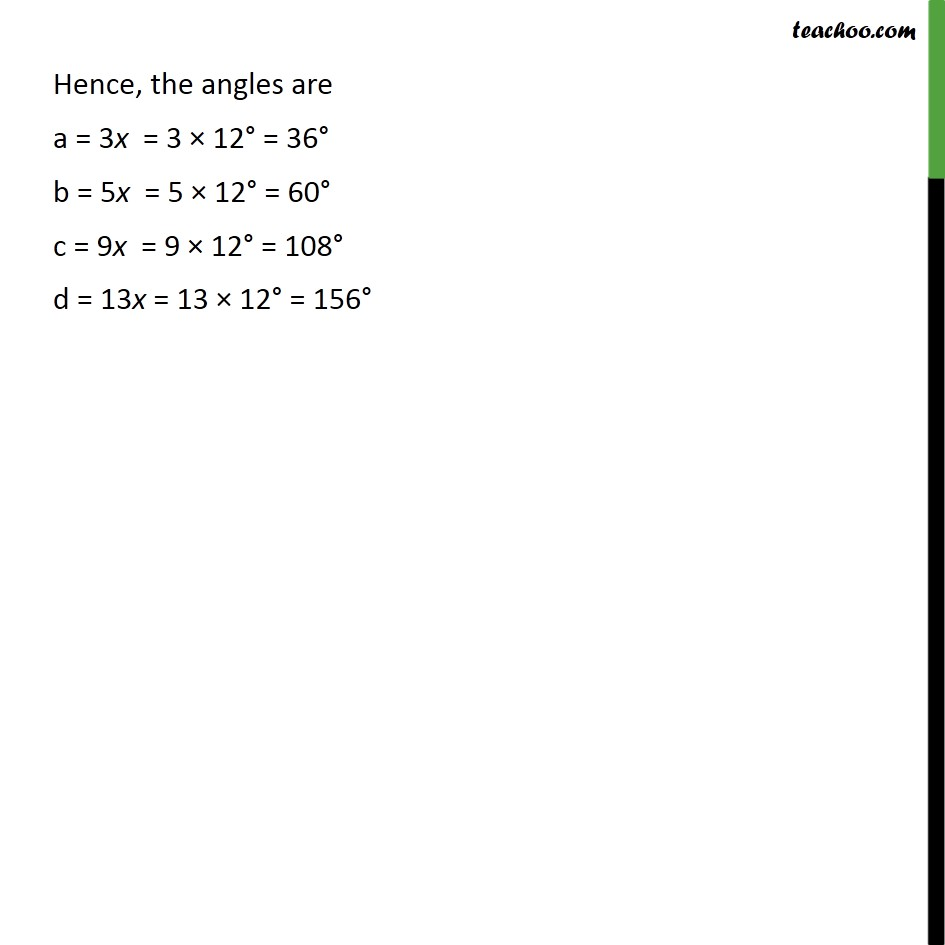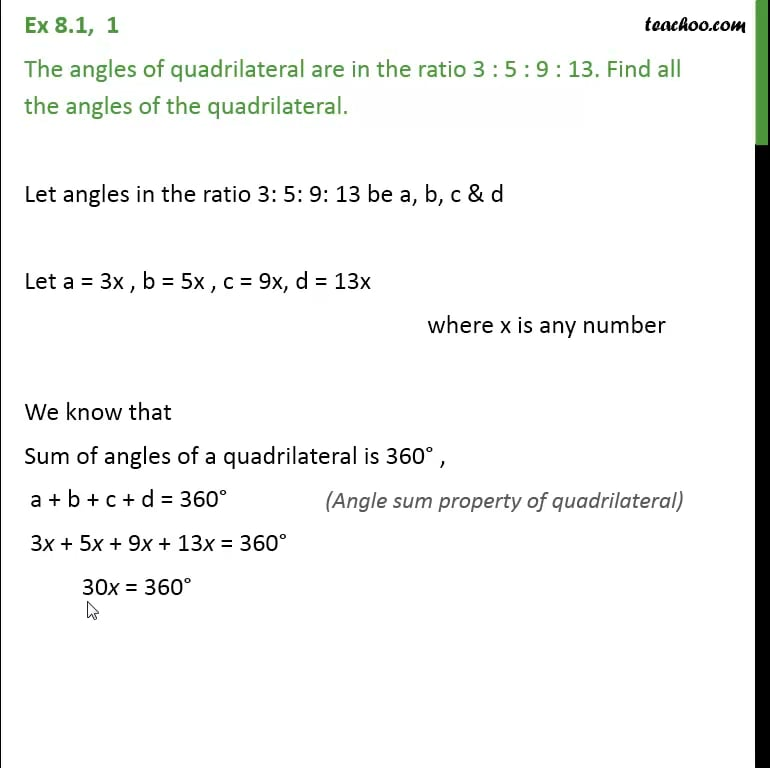Concept wiseThis is only available for Teachoo black users

Learn in your speed, with individual attention - Teachoo Maths 1-on-1 Class

### Transcript

Ex 8.1, 1 The angles of quadrilateral are in the ratio 3 : 5 : 9 : 13. Find all the angles of the quadrilateral. Let angles in the ratio 3: 5: 9: 13 be a, b, c & d Let a = 3x , b = 5x , c = 9x, d = 13x where x is any number We know that Sum of angles of a quadrilateral is 360° , a + b + c + d = 360° 3x + 5x + 9x + 13x = 360° 30x = 360° x = ("360" °)/"30" x = 12° Hence, the angles are a = 3x = 3 × 12° = 36° b = 5x = 5 × 12° = 60° c = 9x = 9 × 12° = 108° d = 13x = 13 × 12° = 156°